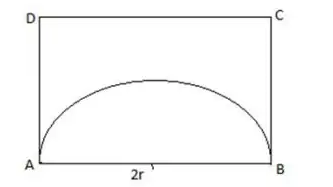# CAT 2018 – Slot 2 – Quantitative Aptitude – The smallest integer n for which 4^n > 17^19 holds

Q. 1: The smallest integer n for which 4^n > 17^19 holds, is closest to?
1. 33
2. 37
3. 39
4. 35

Given , 4^n > 17^19

Or  16^(n/2) > 17^19

Now from the given options, the only possible value of n is 39 as for other

values n/2 will be less than 19 and 16 < 17.

##### Q. 2: The strength of a salt solution is p% if 100 ml of the solution contains p grams of salt. If three salt solutions A, B, C are mixed in the proportion 1 : 2 : 3, then the resulting solution has strength 20%. If instead the proportion is 3 : 2 : 1, then the resulting solution has strength 30%. A fourth solution, D, is produced by mixing B and C in the ratio 2 : 7. The ratio of the strength of D to that of A is1. 2 : 52. 1 : 33. 1 : 44. 3 : 10

let the concentration of A, B & C are a% , b% & c% respectively .

Then (a+2b+3c )/600=20/100=1/5

a+2b+3c=120——1)

And (3a+2b+c )/600=30/100=3/10

3a+2b+c=180——-2)

By eq 1)/ eq 2) , (a+2b+3c )/(3a+2b+c )=2/3

3a+6b+9c=6a+4b+2c

(2b+7c)/a=3

So correct answer : 2.  1 :3

Q. 3: The area of a rectangle and the square of its perimeter are in the ratio 1 : 25. Then the lengths of the shorter and longer sides of the rectangle are in the ratio
1. 1:4
2. 2:9
3. 1:3
4. 3:8

Given ratio of areas of rectangle and square = 1:25 = 4∶ 100=(1×4):(10×10)

Thus possible ratio of perimeter =(1+4)/(10+10) = 5/20= 1∶4

Q. 4: The scores of Amal and Bimal in an examination are in the ratio 11 : 14. After an appeal, their scores increase by the same amount and their new scores are in the ratio 47 : 56. The ratio of Bimal’s new score to that of his original score is
1. 5 : 4
2. 8 : 5
3. 4 : 3
4. 3 : 2

let their scores were 11x and 14x and it increase by n then

(11x+n)/(14x+n)=47/56

616x+56n=658x+47n

42x=9n

n = 42x/9

So Bimal’s new score = 14x + 42x/9 = 168x/9

So required ratio = (168x/9)/14x = 4∶ 3

Q. 5: From a rectangle ABCD of area 768 sq cm, a semicircular part with diameter AB and area 72π sq cm is removed. The perimeter of the leftover portion, in cm, is
1. 80 + 16π
2. 86 + 8π
3. 82 + 24π
4. 88 + 12πGiven area of semicircle = 72π

Or (πr^2)/2 = 72π

r = 12

So AB = 24

So Required perimeter = AD+CD+CB+πr = 32+24+32+12π = 88+12π

##### Checkout Other Questions of CAT 2018 Slot 2 Paper:

Verbal Ability :              |   Q.01- Q.05  |  Q.06- Q.9  |  Q.10- Q.14  |  Q.15- Q.19  |  Q.20- Q.24  |  Q.25- Q.29  |  Q.30 – Q.34  |

Logical Reasoning :    |   Q.29 – Q.32  |

Quantitative Aptitude: |

### Free Material Area